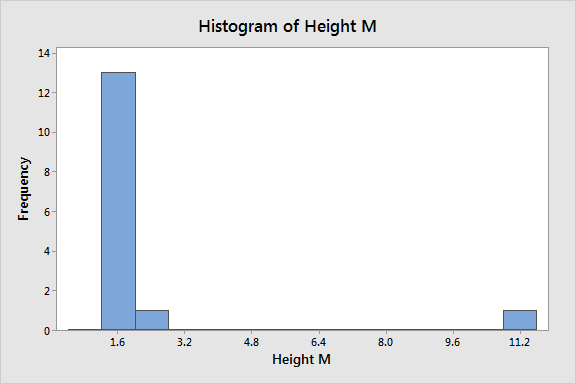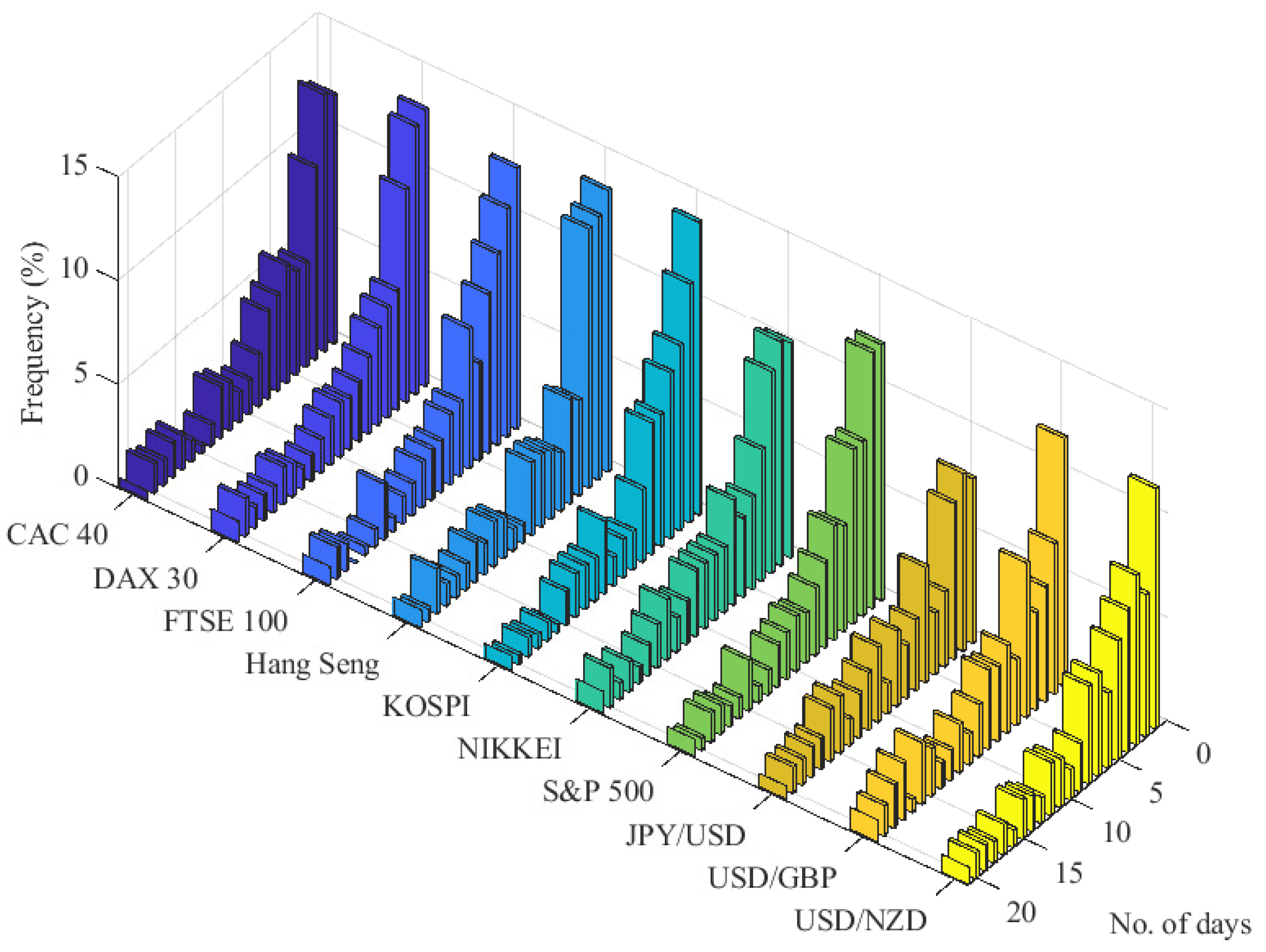# Estimation of Errors

• Astrophysics• Electricity• Electromagnetism• Energy• Fields• Force• Mechanics• Momentum• Nuclear Physics• Quantities & Units• States of Matter• Waves• Key ExperimentsIf you want to know how accurate a measurement is, you need to figure out how much it differs from what you expected to find. There are a few ways to do this, including absolute error, relative error, and percentage error. If you don't have an expected or standard value to compare it to, you can use the average of all your measurements instead. This process is called estimation of errors, and it helps ensure that your data is as reliable as possible.

## The mean value

To calculate the mean, we need to add all measured values of x and divide them by the number of values we took. The formula to calculate the mean is:

Let’s say we have five measurements, with the values 3.4, 3.3, 3.342, 3.56, and 3.28. If we add all these values and divide by the number of measurements (five), we get 3.3764.As our measurements only have two decimal places, we can round this up to 3.38.

## Estimation of errors

Here, we are going to distinguish between estimating the absolute error, the relative error, and the percentage error.

### Estimating the absolute error

To figure out the absolute error of a measurement, you need to compare it to the expected or standard value. For example, if you're measuring the length of a piece of wood and you know it's supposed to be exactly 2.0m long, but your instrument reads 2.003m, your absolute error is 0.003m. This is because you simply subtract the measured value from the expected value to get the difference. It's important to note that the precision of your instrument can affect the accuracy of your measurements.

### Estimating the relative error

To estimate the relative error, we need to calculate the difference between the measured value x0 and the standard value xref and divide it by the total magnitude of the standard value xref. For example, if the measured value is 2.003m and the standard value is 2.0m, then the relative error is | 2.003m-2.0m | / | 2.0m | or 0.00151. As you can see, the relative error is very small and has no units.

### Estimating the percentage error

To estimate the percentage error, we need to calculate the relative error and multiply it by one hundred. The percentage error is expressed as ‘error value’%. For example, if the relative error is 0.0015, then the percentage error is 0.15%. This error tells us the deviation percentage caused by the error.

Using the figures from the previous example, where the measured value is 2.003m and the standard value is 2.0m, the relative error is 0.0015. Multiplying this by 100 gives us a percentage error of 0.15%. This means that the measured value is only off from the standard value by 0.15% or a very small amount.

## What is the line of best fit?

The line of best fit, also known as the regression line, is used when plotting data where one variable depends on another one. By its nature, a variable changes value, and we can measure the changes by plotting them on a graph against another variable such as time. The relationship between two variables will often be linear, meaning that the change in one variable is directly proportional to the change in the other variable.

The line of best fit is the line that is closest to all the plotted values. It is calculated using mathematical formulas that minimize the distance between the line and the plotted points. Some values might be far away from the line of best fit. These are called outliers and may be due to measurement errors or other factors that affect the data.

However, the line of best fit is not a useful method for all data, so we need to know how and when to use it. It is most useful when the data has a linear relationship, meaning that the change in one variable can be accurately predicted by the change in another variable. It can also be used to identify trends and patterns in the data, which can be helpful in making predictions or identifying potential issues.

### Obtaining the line of best fit

To obtain the line of best fit, we need to plot the points as in the example below:

Here, many of our points are dispersed. However, despite this data dispersion, they appear to follow a linear progression. The line that is closest to all those points is the line of best fit.

### When to use the line of best fit

To be able to use the line of best fit, the data need to follow some patterns:

The relationship between the measurements and the data must be linear. The dispersion of the values can be large, but the trend must be clear. The line must pass close to all values.

### Data outliers

Sometimes in a plot, there are values outside the normal range. These are called outliers. If the outliers are fewer in number than the data points following the line, the outliers can be ignored. However, outliers are often linked to errors in the measurements. In the image below, the red point is an outlier.Data plotted from several measurements showing variation on the y axis in black and an outlier in red

### Drawing the line of best fit

To draw the line of best fit, we need to use statistical methods to calculate the slope and intercept of the line that best fits the data. This is done by minimizing the sum of the squared differences between the actual data points and the predicted values on the line.

If the line intersects with the y-axis before the x-axis, the value of y will not necessarily be our minimum value when we measure. The intercept depends on the relationship between the two variables and the range of values that are being measured.

The inclination or slope of the line represents the direct relationship between x and y, and the larger the slope, the steeper the line will be. A large slope means that the data changes very quickly as x increases, while a gentle slope indicates a slower change in the data. However, it is important to note that the slope alone does not provide a complete picture of the relationship between the variables, and other statistical measures may be needed to fully understand the data.

## Calculating uncertainty in a plot

In a plot or a graph with error bars, there can be many lines passing between the bars. We can calculate the uncertainty of the data using the error bars and the lines passing between them. See the following example of three lines passing between values with error bars:Plot showing uncertainty bars and three lines passing between them. The red and green lines begin at the extreme values of the uncertainty bars

Let’s look at an example of this, using temperature vs time data. Calculate the uncertainty of the data in the plot below.Plot showing uncertainty bars and three lines passing between them. The red and green lines begin at the extreme values of the uncertainty bars

To estimate the errors of a measured value, you can compare it to a standard value or reference value. The error can be estimated as an absolute error, a percentage error, or a relative error.

The absolute error measures the total difference between the value you expect from a measurement (X0) and the obtained value (Xref), equal to the absolute value difference of both, Abs = | Xo-Xref |.

The relative and percentage errors measure the fraction of the difference between the expected value and the measured value. In this case, the error is equal to the absolute error divided by the expected value, rel = Abs / Xo for the relative error, and divided by the expected value and expressed as a percentage for the percentage error, per = (Abs / Xo) * 100. It is important to include the percentage symbol for percentage errors.

To approximate the relationship between your measured values, you can use a linear function. This can be done by drawing a line that passes closest to all the values, which is known as the line of best fit. The line of best fit can be useful in identifying trends and making predictions based on the data. However, it is important to use caution when interpreting the results and to consider other statistical measures to fully understand the data.

## Estimation of Errors

What is the best fit line?

The line of best fit is the line that best approaches all data points in a plot, thus serving as an approximation of a linear function to the data.

What does the term ‘error estimation’ mean?

The term ‘error estimation’ refers to the calculation of errors introduced when we measure and use values that have errors in calculations or plots.14-day free trial. Cancel anytime.Join 10,000+ learners worldwide.The first 14 days are on us96% of learners report x2 faster learningFree hands-on onboarding & supportCancel Anytime